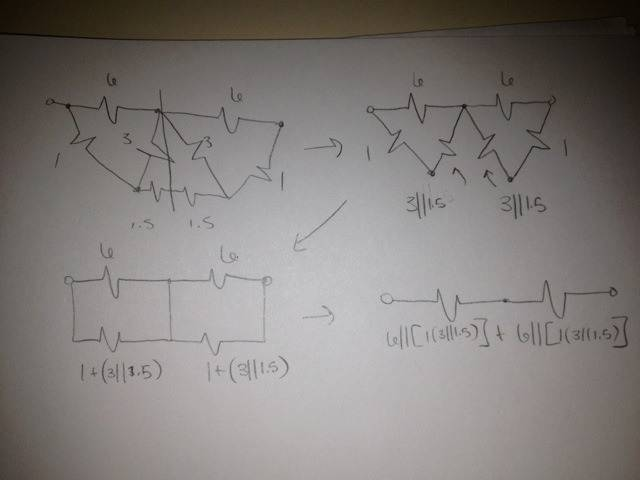# Resistor Circuit

irivceIs it possible to simplify this circuit using just parallel and series definitions ?

* A circuit is parallel to another circuit or several circuits if and only if they share common terminals; i.e. if both the branches touch each other's endpoints

* Resistors can be connected in series; that is, the current flows through them one after another

These are the definitions of resistors in series or parallel and according to these definitions none of these are in series or parallel.This was my attempt at making the circuit a bit easier to simplify but still could not see how any would fit into the definitions.

## Answers and Replies

MentorIs it possible to simplify this circuit using just parallel and series definitions ?

* A circuit is parallel to another circuit or several circuits if and only if they share common terminals; i.e. if both the branches touch each other's endpoints

* Resistors can be connected in series; that is, the current flows through them one after another

These are the definitions of resistors in series or parallel and according to these definitions none of these are in series or parallel.This was my attempt at making the circuit a bit easier to simplify but still could not see how any would fit into the definitions.

Welcome to the PF.

Your 2nd image is missing the 1 Ohm resistor on the right side...

I'm not seeing a way to simplify it offhand, so I would just solve the problem using KCL equations written at the nodes. Can you give that a go to see what answer you get?

Gold Member
2021 Award
look for delta-Y transforms, which is the only alternative to (and derived from) doing your own loop analysis

Saitama
I'm not seeing a way to simplify it offhand..
There is, look at the node between 6 ohm resistors. :)

Gold Member
2021 Award
There is, look at the node between 6 ohm resistors. :)

Uh ... huh?

Saitama
Uh ... huh?

The circuit is symmetrical...can you figure it out now?

Gold Member
2021 Award
Yes, I saw the symmetry but that doesn't seem to eliminate the need to do a delta-Y transform, which is the crux of the OP's problem.

Homework Helper
Gold Member
If you split the "bottom" 3-ohm resistor into two series 1.5-ohms...

Mentor
There is, look at the node between 6 ohm resistors. :)

Interesting. Initially I'd dismissed cutting the circuit in half to use its symmetry, but maybe now I see what you mean. I didn't think cutting the bottom resistor in half bought anything, but I think I see what you're getting at.

Gold Member
2021 Award
If you split the "bottom" 3-ohm resistor into two series 1.5-ohms...

Then you have done nothing, as far as I can see, to avoid the need for a delta-Y transform. I don't see that it buys you anything. Am I missing something. Can you do this without the delta-Y transform (or the equivalent loop analysis) ?

Mentor
Then you have done nothing, as far as I can see, to avoid the need for a delta-Y transform. I don't see that it buys you anything. Am I missing something. Can you do this without the delta-Y transform (or the equivalent loop analysis) ?

Yeah, so there is one more trick to the method that they are proposing. I think we should leave it to the OP to figure out what that trick is. And I think they should also try the wye-delta transformation that you are proposing, to see if he gets the same answer with both techniques (he should).

•1 person
irivce
There is, look at the node between 6 ohm resistors. :)

Alright so I split the 3 ohm resistor, and then could I add a wire to make the 1.5 ohms resitors parallel to the 3 ohm resistors and take it from there ?Saitama
Alright so I split the 3 ohm resistor, and then could I add a wire to make the 1.5 ohms resitors parallel to the 3 ohm resistors and take it from there ? View attachment 61938

Yep!

irivce
Yep!

Awesomee thanks a lot guys!